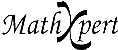# Help With Math: Purveyors of MathXpert

-->## MathXpert: software to help you learn mathematics.### Can MathXpert teach me to do the problems myself?

 MathXpert is based on the following view of mathematics: there are operations that we use to transform mathematical expressions. For example, the operation "collect like terms" can change 3x + 2x to 5x. We solve a problem by applying one operation at a time until our initial problem is transformed to a desired form that we consider the answer. That means there are two parts to learning mathematics: You must learn what the individual operations are and how they work, and you must learn how to decide what operation to apply next. Usually in courses and textbooks, the focus is on the individual operations, but knowing what to do when is often a source of difficulty for students. MathXpert helps you with both parts. First of all, it helps you to understand the individual operations thoroughly. It does this by displaying them explicitly on menus, so that you can see exactly what operations are available. Then, by applying them in examples, you will get a thorough understanding of how they work. Since MathXpert does the actual work of applying them, you won't be troubled by the minor mistakes that so often cause trouble for students. For example, if you apply "collect like terms" to 3x - 2x, you will always get x, never 5x or some other mistaken answer. But perhaps even more important, by using MathXpert to study a particular type of problem (a "topic"), you will learn how to organize several related operations (some of which may be new, and others you are supposed to know already) into a method of solving that type of problem. For instance, you will learn that to solve an equation for x, you need to first get all the occurrences of x on one side of the equation, then combine them to a single x somehow, then manipulate the equation so that one side is exactly x. Just as in the classroom, you learn that by "picking it up" after seeing some examples and then working some examples yourself. What is different about using MathXpert to work the examples is that you have an "expert at your elbow"--your own Algebra Assistant (or Precalculus Assistant or Calculus Assistant) to advise you upon request. Even if you don't request help with the problem-solving strategy, MathXpert still makes sure that you apply each operation correctly: you can never take a mathematically incorrect step.